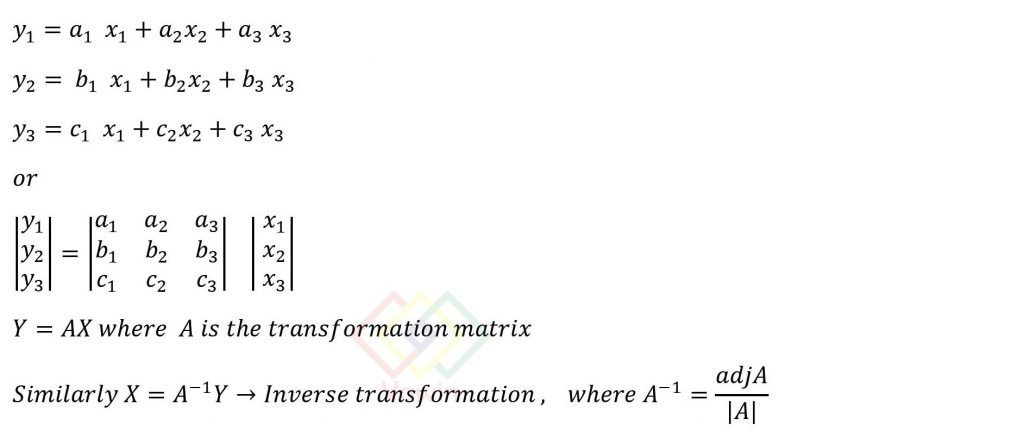## Linear Transformation

Consider a 3 dimension system. Linear transformation in 3D along with its matrix form is given byNote: If Y=AX and Z=BY,then Z=(BA) X=Composite Linear Transformation.

## Topic

Linear Transformation